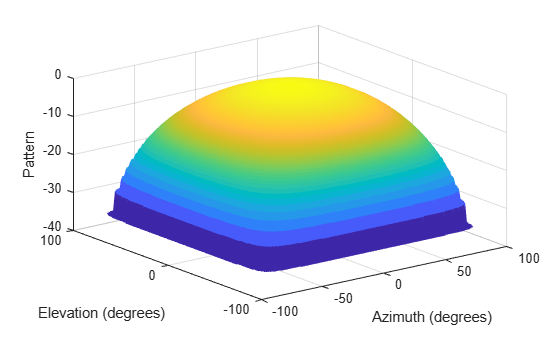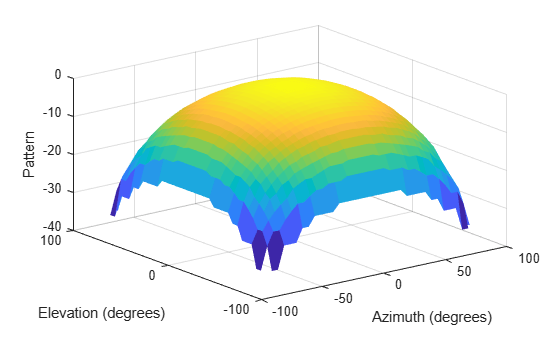Documentation

### This is machine translation

Mouseover text to see original. Click the button below to return to the English version of the page.

# phitheta2azelpat

Convert radiation pattern from phi/theta form to azimuth/elevation form

## Syntax

``pat_azel = phitheta2azelpat(pat_phitheta,phi,theta)``
``pat_azel = phitheta2azelpat(pat_phitheta,phi,theta,az,el)``
``````[pat_azel,az_pat,el_pat] = phitheta2azelpat(___)``````

## Description

example

````pat_azel = phitheta2azelpat(pat_phitheta,phi,theta)` expresses the antenna radiation pattern `pat_phitheta` in azimuth/elevation angle coordinates instead of φ/θ angle coordinates. `pat_phitheta` samples the pattern at φ angles in `phi` and θ angles in `theta`. The `pat_azel` matrix uses a default grid that covers azimuth values from –90 to 90 degrees and elevation values from –90 to 90 degrees. In this grid, `pat_azel` is uniformly sampled with a step size of 1 for azimuth and elevation. The function interpolates to estimate the response of the antenna at a given direction.```

example

````pat_azel = phitheta2azelpat(pat_phitheta,phi,theta,az,el)` uses vectors `az` and `el` to specify the grid at which to sample `pat_azel`. To avoid interpolation errors, `az` should cover the range [–180, 180] and `el` should cover the range [–90, 90].```

example

``````[pat_azel,az_pat,el_pat] = phitheta2azelpat(___)``` returns vectors containing the azimuth and elevation angles at which `pat_azel` samples the pattern, using any of the input arguments in the previous syntaxes.```

## Examples

collapse all

Convert a radiation pattern to azimuth/elevation form, with the azimuth and elevation angles spaced 1° apart.

Define the pattern in terms of φ and θ.

```phi = 0:360; theta = 0:180; pat_phitheta = mag2db(repmat(cosd(theta)',1,numel(phi)));```

Convert the pattern to azimuth/elevation space.

`pat_azel = phitheta2azelpat(pat_phitheta,phi,theta);`

Convert a radiation pattern from theta/phi coordinates to azimuth/elevation coordinates, with azimuth and elevation angles spaced ${1}^{\circ }$ apart.

Define the pattern in terms of phi, $\varphi$, and theta, $\theta$, coordinates.

```phi = 0:360; theta = 0:180; pat_phitheta = mag2db(repmat(cosd(theta)',1,numel(phi)));```

Convert the pattern to azimuth/elevation coordinates. Get the azimuth and elevation angles for use in plotting.

`[pat_azel,az,el] = phitheta2azelpat(pat_phitheta,phi,theta);`

```H = surf(az,el,pat_azel); H.LineStyle = 'none'; xlabel('Azimuth (degrees)'); ylabel('Elevation (degrees)'); zlabel('Pattern');```Convert a radiation pattern from phi/theta coordinates to azimuth/elevation coordinates, with the azimuth and elevation angles spaced ${5}^{\circ }$ apart.

Define the pattern in terms of phi and theta.

```phi = 0:360; theta = 0:180; pat_phitheta = mag2db(repmat(cosd(theta)',1,numel(phi)));```

Define the set of azimuth and elevation angles at which to sample the pattern. Then, convert the pattern.

```az = -180:5:180; el = -90:5:90; pat_azel = phitheta2azelpat(pat_phitheta,phi,theta,az,el);```

```H = surf(az,el,pat_azel); H.LineStyle = 'none'; xlabel('Azimuth (degrees)'); ylabel('Elevation (degrees)'); zlabel('Pattern');```## Input Arguments

collapse all

Antenna radiation pattern in phi/theta form, specified as a Q-by-P matrix. `pat_phitheta` samples the 3-D magnitude pattern in decibels, in terms of φ and θ angles. P is the length of the `phi` vector, and Q is the length of the `theta` vector.

Data Types: `double`

Phi angles at which `pat_phitheta` samples the pattern, specified as a vector of length P. Each φ angle is in degrees, between 0 and 360.

Data Types: `double`

Theta angles at which `pat_phitheta` samples the pattern, specified as a vector of length Q. Each θ angle is in degrees, between 0 and 180.

Data Types: `double`

Azimuth angles at which `pat_azel` samples the pattern, specified as a vector of length L. Each azimuth angle is in degrees, between –180 and 180.

Data Types: `double`

Elevation angles at which `pat_azel` samples the pattern, specified as a vector of length M. Each elevation angle is in degrees, between –90 and 90.

Data Types: `double`

## Output Arguments

collapse all

Antenna radiation pattern in azimuth/elevation form, returned as an M-by-L matrix. `pat_azel` samples the 3-D magnitude pattern in decibels, in terms of azimuth and elevation angles. L is the length of the `az` vector, and M is the length of the `el` vector.

Azimuth angles at which `pat_azel` samples the pattern, returned as a vector of length L. Angles are expressed in degrees.

Elevation angles at which `pat_azel` samples the pattern, returned as a vector of length M. Angles are expressed in degrees.

collapse all

### Phi Angle, Theta Angle

The φ angle is the angle from the positive y-axis toward the positive z-axis, to the vector’s orthogonal projection onto the yz plane. The φ angle is between 0 and 360 degrees. The θ angle is the angle from the x-axis toward the yz plane, to the vector itself. The θ angle is between 0 and 180 degrees.

The figure illustrates φ and θ for a vector that appears as a green solid line. The coordinate system is relative to the center of a uniform linear array, whose elements appear as blue circles.The coordinate transformations between φ/θ and az/el are described by the following equations

`$\begin{array}{l}\mathrm{sin}\left(\text{el}\right)=\mathrm{sin}\varphi \mathrm{sin}\theta \hfill \\ \mathrm{tan}\left(\text{az}\right)=\mathrm{cos}\varphi \mathrm{tan}\theta \hfill \\ \hfill \\ \mathrm{cos}\theta =\mathrm{cos}\left(\text{el}\right)\mathrm{cos}\left(\text{az}\right)\hfill \\ \mathrm{tan}\varphi =\mathrm{tan}\left(\text{el}\right)/\mathrm{sin}\left(\text{az}\right)\hfill \end{array}$`

### Azimuth Angle, Elevation Angle

The azimuth angle of a vector is the angle between the x-axis and the orthogonal projection of the vector onto the xy plane. The angle is positive in going from the x axis toward the y axis. Azimuth angles lie between –180 and 180 degrees. The elevation angle is the angle between the vector and its orthogonal projection onto the xy-plane. The angle is positive when going toward the positive z-axis from the xy plane. These definitions assume the boresight direction is the positive x-axis.

### Note

The elevation angle is sometimes defined in the literature as the angle a vector makes with the positive z-axis. The MATLAB® and Phased Array System Toolbox™ products do not use this definition.

This figure illustrates the azimuth angle and elevation angle for a vector that appears as a green solid line. The coordinate system is relative to the center of a uniform linear array, whose elements appear as blue circles.